# 前言

``````double money = 1.0 - 0.9;

# 解决方案

## Decimal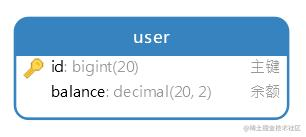``````BigDecimal d1 = new BigDecimal(0.1); // BigDecimal(double val)
BigDecimal d2 = new BigDecimal("0.1"); // BigDecimal(String val)
BigDecimal d3 = BigDecimal.valueOf(0.1); // static BigDecimal valueOf(double val)

``````d1: 0.1000000000000000055511151231257827021181583404541015625
d2: 0.1
d3: 0.1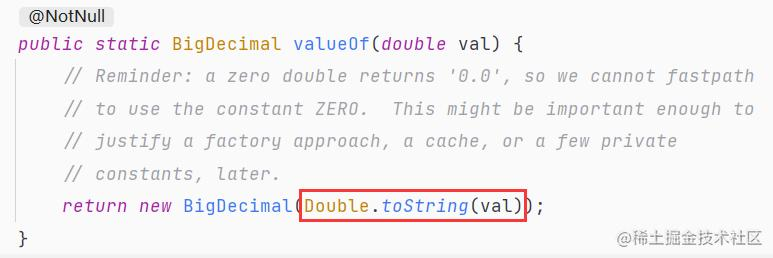``````BigDecimal add(BigDecimal); // 加
BigDecimal subtract(BigDecimal); // 减
BigDecimal multiply(BigDecimal); // 乘
BigDecimal divide(BigDecimal); // 除

`BigDecimal` 是不可变对象，意思就是这些操作都不会改变原有对象的值，方法执行完毕只会返回一个新的对象。若要运算后更新原有值，只能重新赋值：

``````d1 = d1.subtract(d2);

``````BigDecimal d1 = new BigDecimal("1.0");
BigDecimal d2 = new BigDecimal("0.9");
System.out.println(d1.subtract(d2));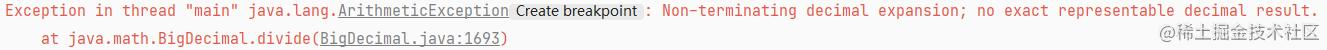``````BigDecimal divide(BigDecimal divisor, int scale, int roundingMode)

`scale` 参数表示运算后保留几位小数，`roundingMode` 参数表示计算小数的方式。

``````BigDecimal d1 = new BigDecimal("1.0");
BigDecimal d2 = new BigDecimal("3");
System.out.println(d1.divide(d2, 2, RoundingMode.DOWN)); // 小数精度为2，多余小数直接舍去。输出结果为0.33

`RoundingMode` 枚举能够方便地指定小数运算方式，除了直接舍去，还有四舍五入、向上取整等多种方式，根据具体业务需求指定即可。

``````BigDecimal d1 = new BigDecimal("0.33");
BigDecimal d2 = new BigDecimal("0.3300");
System.out.println(d1.equals(d2)); // false

``````BigDecimal d1 = new BigDecimal("0.33");
BigDecimal d2 = new BigDecimal("0.3300");
System.out.println(d1.compareTo(d2) == 0); // true

`d1` 大于 `d2`，返回 `1`

`d1` 小于 `d2`，返回 `-1`

`BigDecimal` 的用法就介绍到这，我们接下来看第二种解决方案。

## 定长整数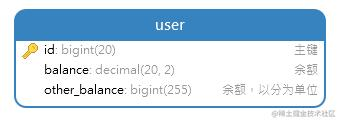``````long d1 = 10000L; // 100元
d1 += 500L; // 加五元
d1 -= 500L; // 减五元

``````long d1 = 2366L; // 23.66元
double result = d1 * 0.8; // 打八折，运算后结果为1892.8
d1 = (long)result; // 转换为整数，舍去所有小数，值为1892。即18.92元

``````long d1 = 2366L; // 23.66元
double result = d1 * 0.8; // 运算后结果为1892.8
d1 = (long)result; // 强转舍去所有小数，值为1892
d1 = (long)Math.ceil(result); // 向上取整，值为1893
d1 = (long)Math.round(result); // 四舍五入，值为1893
...

``````long d1 = 2366L;
long result = d1 / 3; // 正确的值本应该为788.6666666666666，舍去所有小数，最终值为788

``````long d1 = 2366L;
double result = d1 / 3.0; // 注意，这里除以不是 3，而是 3.0 浮点数
d1 = (long)Math.round(result); // 四射勿入，最终值为789，即7.89元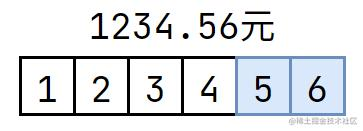• Decimal 类型
• 定长整数